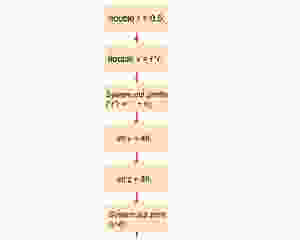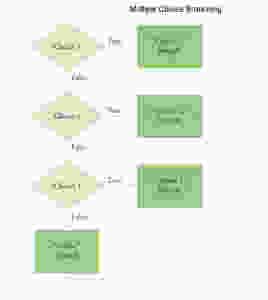Questions? Email

# 5.1 - What is the third and last type of flow control?

Edited: 6 days ago

A. Introduction

There are probably millions of programs in the world now, but all programs are made of three different "flows", or control structures: sequential, branching and looping.

Sequential execution goes from top to bottom, line by line.Branching execution allows you to make decisions based on a condition:You can even embed a branching structure in one branch:Or, making one decision right after another:B. So what's the third and last type of flow (control structure)?

C. Try to write some pseudo code to add all even numbers between 200 and 300.

New Posts
• ## 5.2 - How to repeat some actions N times?

A. Introduction The third and last type of flow is all about repetitions: how to repeat some actions exact N times? or how to repeat some actions until certain status is achieved? There are actually three types of statements: for loops, while loops and do loops. B. Watch( Slides ) C. Try: Print out a multiplication table from 1 x 1 = 1 .... to 9 x 9 = 81. (Hint: you need a for loop inside a for loop)
• ## 5.3 - How to print out a triangular multiplication table?

A. Introduction You were asked to use for-loops to print out a multiplication table, for-loops and nested for-loops are widely used for many applications. Here are some examples. B. Watch( Slides ) C. Try to print out the upper half or the lower half of a multiplication table like this:
• ## 5.7 What's the difference between do...while and while?

A. Introduction do loops are essentially same at while loops except that they will run at least once. All three types of loops can be converted into each other... Which one to choose? B. Watch( slides ) C. Try this: Write a do loop that reads integers and computes their sum. Stop when reading a zero or the same value twice in a row. For example, if the input is 1 2 3 4 4, then the sum is 14 and the loop stops.